# JS学习：JavaScript的核心发布者 ourjs  发布时间 1385940964000

# 对象

ECMAScript做为一个高度抽象的面向对象语言，是通过对象来交互的。即使ECMAScript里边也有基本类型，但是，当需要的时候，它们也会被转换成对象。

``````一个对象就是一个属性集合，并拥有一个独立的prototype（原型）对象。这个prototype可以是一个对象或者null。
``````

``````var foo = {
x: 10,
y: 20
};
``````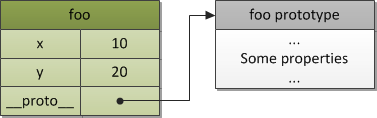# 原型链

``````原型链是一个用来实现继承和共享属性的有限对象链。
``````

ECMAScript中没有类的概念。但是，代码重用的风格并没有太多不同（尽管从某些方面来说比基于类（class-based）的方式要更加灵活）并且通过原型链来实现。这种继承方式叫作委托继承(delegation based inheritance)（或者，更贴近ECMAScript一些，叫作原型继承(prototype based inheritance)）。

``````var a = {
x: 10,
calculate: function (z) {
return this.x + this.y + z
}
};

var b = {
y: 20,
__proto__: a
};

var c = {
y: 30,
__proto__: a
};

// call the inherited method
b.calculate(30); // 60
c.calculate(40); // 80
``````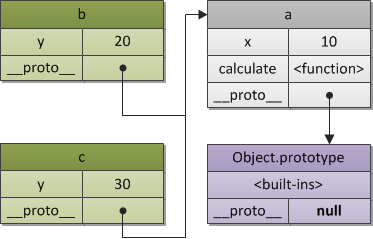``````var b = Object.create(a, {y: {value: 20}});
var c = Object.create(a, {y: {value: 30}});
``````

# 构造函数

``````// a constructor function
function Foo(y) {
// which may create objects
// by specified pattern: they have after
// creation own "y" property
this.y = y;
}

// also "Foo.prototype" stores reference
// to the prototype of newly created objects,
// so we may use it to define shared/inherited
// properties or methods, so the same as in
// previous example we have:

// inherited property "x"
Foo.prototype.x = 10;

// and inherited method "calculate"
Foo.prototype.calculate = function (z) {
return this.x + this.y + z;
};

// now create our "b" and "c"
// objects using "pattern" Foo
var b = new Foo(20);
var c = new Foo(30);

// call the inherited method
b.calculate(30); // 60
c.calculate(40); // 80

// let's show that we reference
// properties we expect

console.log(

b.__proto__ === Foo.prototype, // true
c.__proto__ === Foo.prototype, // true

// also "Foo.prototype" automatically creates
// a special property "constructor", which is a
// reference to the constructor function itself;
// instances "b" and "c" may found it via
// delegation and use to check their constructor

b.constructor === Foo, // true
c.constructor === Foo, // true
Foo.prototype.constructor === Foo // true

b.calculate === b.__proto__.calculate, // true
b.__proto__.calculate === Foo.prototype.calculate // true

);
``````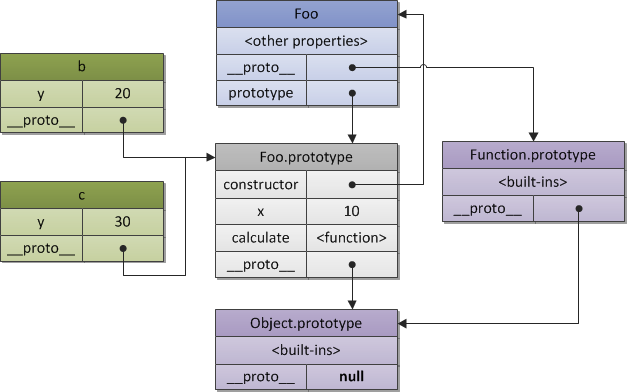``````// ES6
class Foo {
constructor(name) {
this._name = name;
}

getName() {
return this._name;
}
}

class Bar extends Foo {
getName() {
return super.getName() + ' Doe';
}
}

var bar = new Bar('John');
console.log(bar.getName()); // John Doe
``````

# 执行上下文堆栈

``````function foo(bar) {}

// call the same function,
// generate three different
// contexts in each call, with
// different context state (e.g. value
// of the "bar" argument)

foo(10);
foo(20);
foo(30);
``````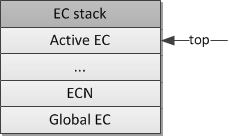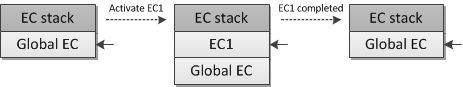# 执行上下文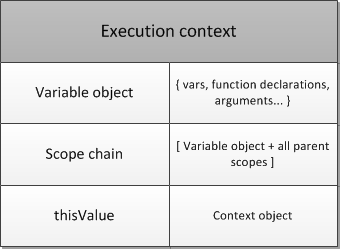# 变量对象

``````变量对象是与执行上下文相关的数据作用域。它是一个与上下文相关的特殊对象，其中存储了在上下文中定义的变量和函数声明。
``````

``````var foo = 10;

function bar() {} // function declaration, FD
(function baz() {}); // function expression, FE

console.log(
this.foo == foo, // true
window.bar == bar // true
);

console.log(baz); // ReferenceError, "baz" is not defined
``````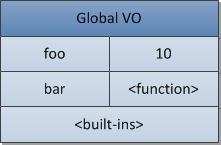# 活动对象

``````function foo(x, y) {
var z = 30;
function bar() {} // FD
(function baz() {}); // FE
}

foo(10, 20);
``````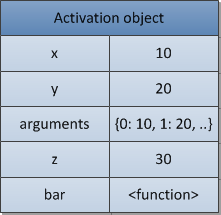# 作用域链

``````作用域链是一个对象列表，上下文代码中出现的标识符在这个列表中进行查找。
``````

``````var x = 10;

(function foo() {
var y = 20;
(function bar() {
var z = 30;
// "x" and "y" are "free variables"
// and are found in the next (after
// bar's activation object) object
// of the bar's scope chain
console.log(x + y + z);
})();
})();
````````````Object.prototype.x = 10;

var w = 20;
var y = 30;

// in SpiderMonkey global object
// i.e. variable object of the global
// context inherits from "Object.prototype",
// so we may refer "not defined global
// variable x", which is found in
// the prototype chain

console.log(x); // 10

(function foo() {

// "foo" local variables
var w = 40;
var x = 100;

// "x" is found in the
// "Object.prototype", because
// {z: 50} inherits from it

with ({z: 50}) {
console.log(w, x, y , z); // 40, 10, 30, 50
}

// after "with" object is removed
// from the scope chain, "x" is
// again found in the AO of "foo" context;
// variable "w" is also local
console.log(x, w); // 100, 40

// and that's how we may refer
// shadowed global "w" variable in
// the browser host environment
console.log(window.w); // 20

})();
``````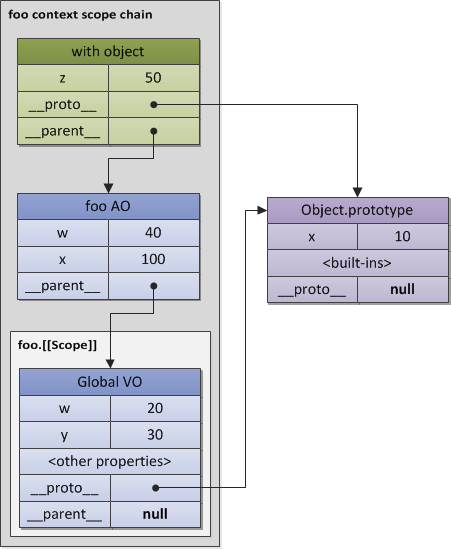# 闭包

「funarg问题」的第一个子问题是「向上funarg问题」（upward funarg problem）。它会在当一个函数从另一个函数向上返回（到外层）并且使用上面所提到的自由变量的时候出现。为了在即使父函数上下文结束的情况下也能访问其中的变量，内部函数在被创建的时候会在它的`[[Scope]]`属性中保存父函数的作用域链。所以当函数被调用的时候，它上下文的作用域链会被格式化成活动对象与`[[Scope]]`属性的和（实际上就是我们刚刚在上图中所看到的）：

``````Scope chain = Activation object + [[Scope]]
``````

``````function foo() {
var x = 10;
return function bar() {
console.log(x);
};
}

// "foo" returns also a function
// and this returned function uses
// free variable "x"

var returnedFunction = foo();

// global variable "x"
var x = 20;

// execution of the returned function

returnedFunction(); // 10, but not 20
``````

「funarg问题」的第二个部分是「向下funarg问题」。这种情况下可能会存在一个父上下文，但是在解析标识符的时候可能会模糊不清。问题是：标识符该使用哪个作用域的值－以静态的方式存储在函数创建时刻的还是在执行过程中以动态方式生成的（比如caller的作用域）？为了避免这种模棱两可的情况并形成闭包，静态作用域被采用：

``````// global "x"
var x = 10;

// global function
function foo() {
console.log(x);
}

(function (funArg) {

// local "x"
var x = 20;

// there is no ambiguity,
// because we use global "x",
// which was statically saved in
// [[Scope]] of the "foo" function,
// but not the "x" of the caller's scope,
// which activates the "funArg"

funArg(); // 10, but not 20

})(foo); // pass "down" foo as a "funarg"
``````

``````闭包是一个代码块（在ECMAScript是一个函数）和以静态方式/词法方式进行存储的所有父作用域的一个集合体。所以，通过这些存储的作用域，函数可以很容易的找到自由变量。
``````

``````function baz() {
var x = 1;
return {
foo: function foo() { return ++x; },
bar: function bar() { return --x; }
};
}

var closures = baz();

console.log(
closures.foo(), // 2
closures.bar()  // 1
);
``````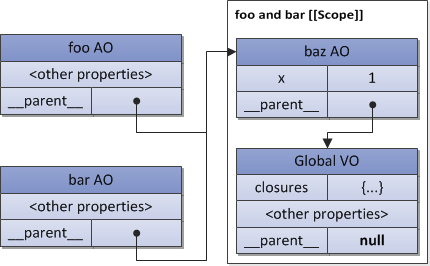``````var data = [];

for (var k = 0; k < 3; k++) {
data[k] = function () {
};
}

data(); // 3, but not 0
data(); // 3, but not 1
data(); // 3, but not 2
``````

``````var data = [];

for (var k = 0; k < 3; k++) {
data[k] = (function (x) {
return function () {
};
})(k); // pass "k" value
}

// now it is correct
data(); // 0
data(); // 1
data(); // 2
``````

# This

``````this是一个与执行上下文相关的特殊对象。因此，它可以叫作上下文对象（也就是用来指明执行上下文是在哪个上下文中被触发的对象）。
``````

``````this是执行上下文的一个属性，而不是变量对象的一个属性
``````

``````var x = 10;

console.log(
x, // 10
this.x, // 10
window.x // 10
);
``````

``````// the code of the "foo" function
// never changes, but the "this" value
// differs in every activation

function foo() {
}

// caller activates "foo" (callee) and
// provides "this" for the callee

foo(); // global object
foo.prototype.constructor(); // foo.prototype

var bar = {
baz: foo
};

bar.baz(); // bar

(bar.baz)(); // also bar
(bar.baz = bar.baz)(); // but here is global object
(bar.baz, bar.baz)(); // also global object
(false || bar.baz)(); // also global object

var otherFoo = bar.baz;
otherFoo(); // again global object
``````

# 总结

##### 开源的 OurJS
OurJS开源博客已经迁移到 OnceOA 平台。

##### 关注我们

OnceJS